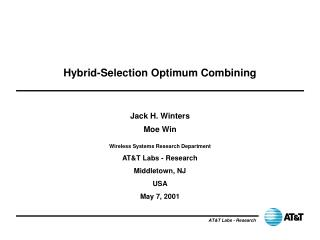DownloadDownload PresentationHybrid-Selection Optimum Combining

# Hybrid-Selection Optimum Combining

Download Presentation## Hybrid-Selection Optimum Combining

- - - - - - - - - - - - - - - - - - - - - - - - - - - E N D - - - - - - - - - - - - - - - - - - - - - - - - - - -
##### Presentation Transcript

1. Hybrid-Selection Optimum Combining Jack H. Winters Moe Win AT&T Labs - Research Middletown, NJ USA May 7, 2001

2. OUTLINE • Introduction • Single Link Results • Cellular Performance • Conclusions

3. W1 Output  Output WM Diversity Techniques Selection: • Select antenna with the highest received signal power Maximal ratio combining: • Weight and combine signals to maximize signal-to-noise ratio (M-fold diversity)

4. W1 Output  WM Diversity Techniques (cont.) Optimum combining: • Weight and combine signals to maximize signal-to- interference-plus-noise ratio (SINR) • Suppresses K<M-1 interferers with M-K diversity gain

5. Hybrid Diversity Techniques • RF chains are the most expensive component • Therefore, select L out of M antennas • H-S/MRC: Select L antennas with the highest S/N and combine with maximal ratio combining • Proposed Technique: Hybrid-Selection/Optimum Combining (H-S/OC) • Select L out of M antennas that maximize SINR with optimum combining

6. H-S/OC Issues • Unlike H-S/MRC, no known technique to find best set of L => exhaustive search of all M!/(L!(M-L)!) combinations required • Need to know interference level => training sequences

7. H-S/OC for L=1 • Selection based on SINR versus S • Rayleigh fading of desired and K equal-power interferers • 5 dB gain for M=10

8. H-S/OC for L>1 • K equal-power interferers • K=L-1: interference suppression with increasing diversity gain as M increases • K=L: for L=M, only a few dB better than MRC, but increasing improvement of H-S/OC over H-S/MRC as M increases • Improvement even for K=L+1

9. Cellular System Results • ANSI-136 model • 98 cells for N=7, 36 cells for N=1,3,4 (3 sectors/cell) • Computer simulation for outage probability (BER > 2 X 10e-2) with 60% loading

10. Uplink Results • 10% outage requires: • L=1: • M=2 for N=3,4 • M=15 for N=1 • L=2: • M=4 for N=1

11. Downlink Results • Results similar to, but slightly better than, uplink results • L=3 performance at: • L=2, M=4 • L=1, M=16 • As L increases, outage decreases faster with M

12. Conclusions • Proposed Hybrid-Selection/Optimum Combining • Suppresses K>L-1 interferers if L<M • Improvement increases with M even for M>>L • For ANSI-136, dramatically reduces outage • 7-fold capacity increase with L=1, M=12 or L=2, M=4 • Suppresses multiple strong interferers and obtains diversity gain with a small number of receiver chains